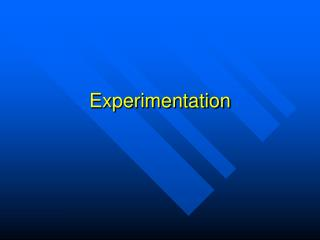DownloadDownload PresentationExperimentation

# Experimentation

Télécharger la présentation## Experimentation

- - - - - - - - - - - - - - - - - - - - - - - - - - - E N D - - - - - - - - - - - - - - - - - - - - - - - - - - -
##### Presentation Transcript

1. Experimentation

2. Variables • Variable = an aspect of events or things that differs according to the particular event or thing being considered but has a particular value for a given event or thing at a given time • Ex. Variable = weight Things = persons Different persons weigh different amounts but a single person weighs a certain amount at a given time.

3. Variables are Pervasive • Every dimension by which an entity or event can be described can be considered a variable.

4. Experiments and Variables • Experiments are conducted to attempt to find out the relationships among variables.

5. Quantifying Variables • Variables can be quantified by measuring their different values.

6. Functional Relationships • Variables are functionally related when the value of one variable determines another. • This can be stated mathematically: Y = f (X). The value of the variable Y is a function of the value of the variable X. • Ex. Running Speed = f (weight carried)

7. Experimental Design • Experiments must be carefully designed to determine the functional relationships among variables. • The influence of variables other than those being tested must be controlled.

8. Experimental Variables • Independent Variables • Dependent Variables • Control Variables

9. Independent Variables • Directly manipulated by the experimenter and determined before the experiment begins • Experiments are designed to see how manipulating the independent variable will effect the dependent variable.

10. Example of an Independent Variable • A runner is clocked sprinting for 100 yards with a backpack 6 times carrying 0, 10, 20, 30, 40 and 50 pounds. • The weight in the backpack is the independent variable

11. Dependent Variables • The values of the dependent variable are the product of the experimental conditions. • They are a function of the values of the independent variable. • Dependent = f (Independent) • They are measured under each of the values of the independent variable.

12. Example of a Dependent Variable • A runner is clocked sprinting for 100 yards with a backpack 6 times carrying 0, 10, 20, 30, 40 and 50 pounds. • The runner’s speed is the dependent variable

13. Control Variables • Control variables are set so that they cannot systematically affect the relationship between the independent and dependent variables. • The average value of a control variable should not change as the independent variable changes.

14. Example of Control Variables • A runner is clocked sprinting for 100 yards with a backpack 6 times carrying 0, 10, 20, 30, 40 and 50 pounds. • The time that the 6 races are run is a control variable and should be set so that the runner doesn’t get more and more tired with each race.

15. Ways of Setting Control Variables • Have no variation of the control variable among different experimental conditions. • Have the same distribution of the control variable among the different experimental conditions. • Have the values of the control variable randomly assigned among the different experimental conditions.

16. Variables and Hypotheses • Experimental scientists must try to relate some terms of a hypothesis to dependent variables and some of them to independent variables.

17. Hypothesis Testing • A well designed experiment will permit observations under controlled conditions and reveal the relationship between independent and dependent variables and thus of the hypothetical terms.